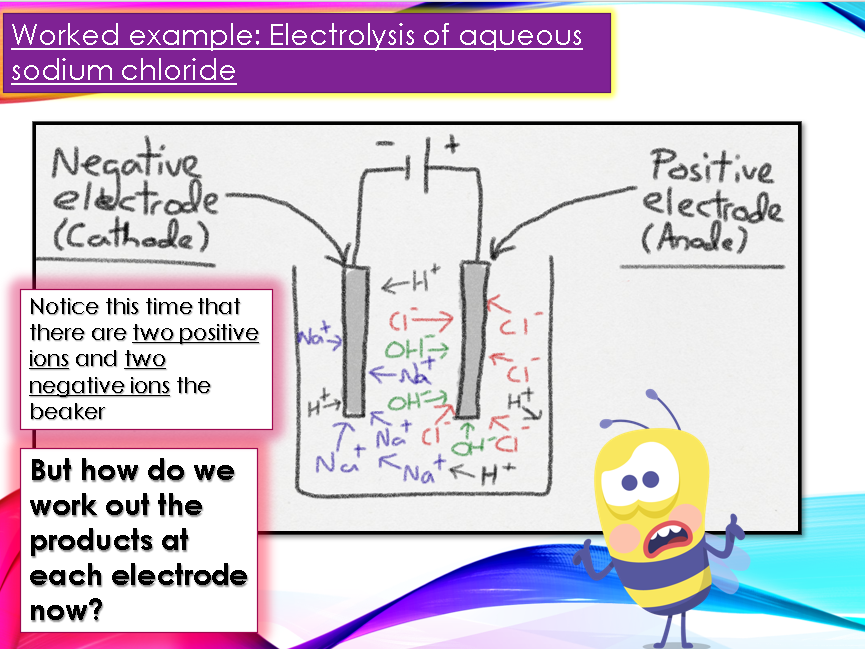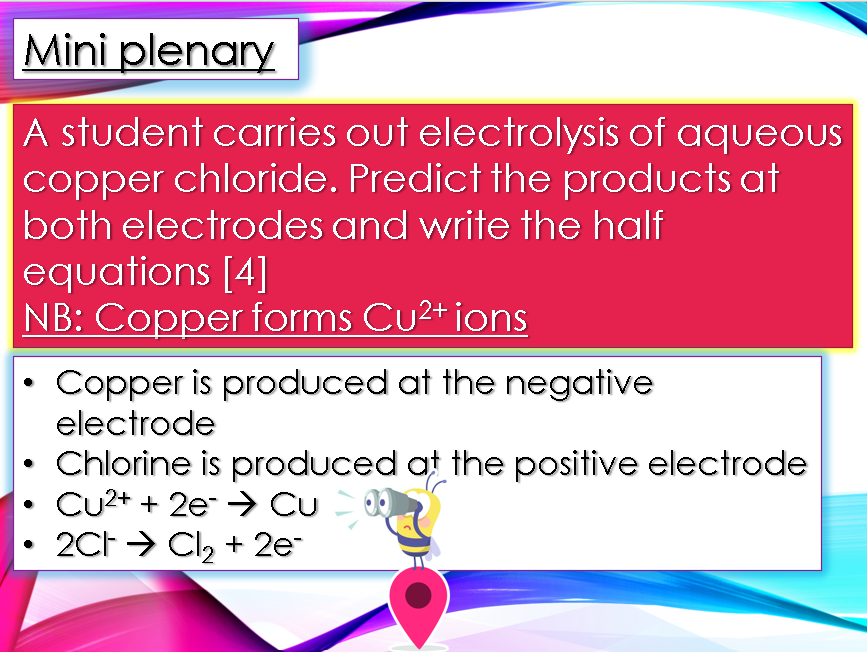Last updated

29 April 2021

#### Share thispptx, 4.08 MB

Electrolysis of aqueous solutions and electrolysis of ionic compounds with half equations. Lesson objectives: Predict the products of the electrolysis of binary ionic compounds in the molten state, write half equations for the reactions occurring at the electrodes during electrolysis and predict the products of the electrolysis of aqueous solutions containing a single ionic compound. This lesson follows on from the introduction lesson to electrolysis that can be found here. All the slides in this lesson are fully animated and include answers to every mini plenary question and exam question. The breakdown of the slides is as follows:

Slide 1 - Title and 5-minute starter. The starter is a grid of four questions entitled ‘last week, last lesson, today’s learning and future learning’. Use this generic slide for all of your lessons by simply changing the questions and the answers each time.
Slide 2 - Lesson objectives (see above)
Slide 3 – Recap slide from previous lesson on what happens when an ionic compound is melted or dissolved in water, i.e. the ions are free to move about within the liquid or solution
Slide 4 – An explanation of what you would see at each electrode during the electrolysis of molten sodium chloride, i.e. bubbles of chlorine gas at positive electrode and sodium metal coating the negative electrode
Slide 5 – Mini plenary/learning pit stop. Three questions of increasing difficulty to check students’ understanding thus far. Answers animate onto the screen.
Slide 6 – Introduction to half equations, the golden rule (Positive ions gain electrons to form elements and negative ions lose electrons to form elements) and OIL RIG all covered here
Slide 7 – Worked example of electrolysis of molten sodium chloride. Half equations animate onto the screen
Slide 8 – List of diatomic elements presented to students in the form of useful mnemonic (Have No Fear Of Ice Cold Beer)
Slide 9 – Exam-style question worth 4 marks: A student performs electrolysis of molten magnesium chloride. Predict the products at each electrode and write half equations for each electrode. Answers animate onto the screen as you click the mouse.
Slide 10 – Introduction to the electrolysis of aqueous solutions with mention of hydroxide and hydrogen ions from the water
Slide 11 – Diagram of electrolysis of aqueous sodium chloride solution showing all the ions present
Slide 12 – Golden rules to help students work out the products at each electrode: Hydrogen is produced at the negative electrode IF the metal is more reactive than hydrogen and Oxygen is produced at the positive electrode unless the solution contains halide ions, in which case the halogen is produced.
Slide 13 – Solutions given for the products at each electrode for the electrolysis of aqueous sodium chloride solution using the golden rules
Slide 14 – Special mention for the half equation for the oxidation of hydroxide ions to form oxygen and water, as this one is particularly difficult.
Slide 15 - Exam

### Review

5

Something went wrong, please try again later.

#### Mr_Mendeleev

a month ago
5

I used this lesson with my top set Y1O and it was amazing! My school uses AQA Trilogy and this lesson seemed to cover all the spec points.

Report this resourceto let us know if it violates our terms and conditions.
Our customer service team will review your report and will be in touch.

£3.00